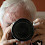## Saturday, April 14, 2012

### Probability, The Number e, and Magic all in one

Since I have not done any mathematical magic posts recently, I wanted to make sure we got one in.
Here is a fun trick you can do for your friends that is all based on simple math. Ask them what the odds would be that if you give them a card in the deck, they could guess the value of the card. They should say one in thirteen, or about eight percent.
What about guessing the value wrong. That would be 92%.
Challenge them to get through the whole entire deck and get every single card’s value wrong. Pretty easy right, you only have an eight percent chance of getting it right. Watch their stunned reaction when they get halfway through and get one correct.
The Method: As I said, it is all based on mathematics. To calculate the odds, you must multiply together the odds of getting it wrong every single time. So, you would have to multiply the .92 by itself fifty-two times. This gives you:
(12/13)^52 = .0183...
Basically, there is a 1.83% chance that you will get through the deck getting it wrong every time. I thought that was pretty cool.
If you’re in a mathematical mood, it gets even cooler. Say you did it with the full card rather than just the value. This would give you:
(51/52)^52 = .3643...
Still good odds. But check this out. Remember back when we studied the number e? Well, what is the reciprocal of e, or 1/e?
1/e = .3678...
They aren’t exact, but that is an extremely close estimate, right! This is true because of our formula for e^x. Let’s go back to it.
e^x ≈ (1 + x/n)^n
In this case, 51/52 would be written as:
(1 + -1/52)^52
With this rule, this should be about e^-1, which means the same thing as the reciprocal of e.
You could use this same logic to figure it out for just the value. (12/13)^52 is the same as:
(1 + -4/52)^52
This means that the odds are approximately e^-4, which is 1/e^4. I thought that this trick is cool, but the reasoning behind it is even cooler.

1.Nice blog and I Want to give some suggestions in solving percent word problems.It has three numbers-
One is the base B which represents the total amount of something or give the measure of something.
Second is the rate R which is expressed in percents, like 3%, 7.5%, 12.75%.
Third is the part P. It is the amount or the measure of the part.
Algebra Word Problems Worksheet

2.begin pedant mode
I think you need more details in the game rules, e.g., never mention the same card more than once or give a full list for the entire deck in advance. Otherwise one would get a 51/52 change of winning your game by calling a random card and then (if there is a "then") calling whatever card came up first.
end pedant mode

3.begin even more pedant mode
Forbidding to mention each card more then once does not help. You can just mention the last card in each turn after the first one. Once the card you guessed as first card comes up, you guess a random one of the remaining cards. Not exactly 51/52, but better (ehm, worse) than average guessing.
end even more pedant mode